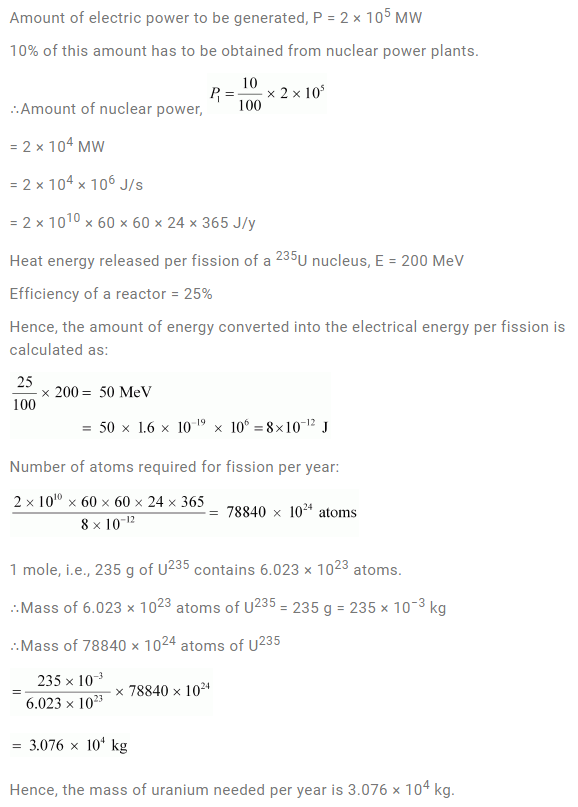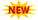SSC Phase 10 Notification || TSSPDCL AE Notification || PPSC Naib Tehsildar Admit Card ||

# NCERT Solutions For Class 12 Physics Chapter 13 Nuclei

NCERT Solutions For Class 12 Physics Chapter 13 mainly comprises solutions for problems based on radioactive decay such as Alpha Decay, Beta Decay, Gamma Decay, etc. With the help of Nuclei Class 12 NCERT Solutions given in this article, students can better understand and solve the problems at the end of the lesson. This chapter has mainly about constituents of the atomic nuclei, such as Proton and Neutron, how they were discovered by different scientists, Radiation, the Half-life of radioactive elements, and Radio-Activity. Solving through the problems given at the back of the chapter and referring the NCERT Solutions For Physics for guidance on tough ones enables the students to get good marks in board exam as well as in competitive examinations. NCERT Solutions page contains links for more solutions and different classes. To understand how nuclear reactors work, Nuclear Fission and Nuclear Fusion, Control Rods, production of electricity with its help, aspirants have to read this chapter well.

## Class 12 Physics Nuclei NCERT Solutions

Subtopics in the NCERT Solutions For Class 12 Physics Chapter 13 are given below:

 Section Topic Name 13 Nuclei 13.1 Introduction 13.2 Atomic Masses and Composition of Nucleus 13.3 Size of the Nucleus 13.4 Mass-Energy and Nuclear Binding Energy 13.5 Nuclear Force 13.6 Radioactivity 13.7 Nuclear Energy

### Class 12 Physics Chapter 13 Nuclei NCERT Solutions

Question 13.1: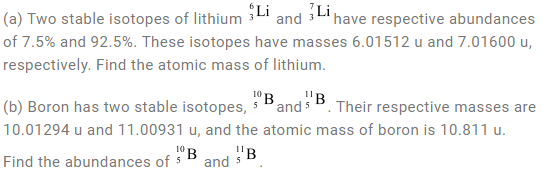Solution: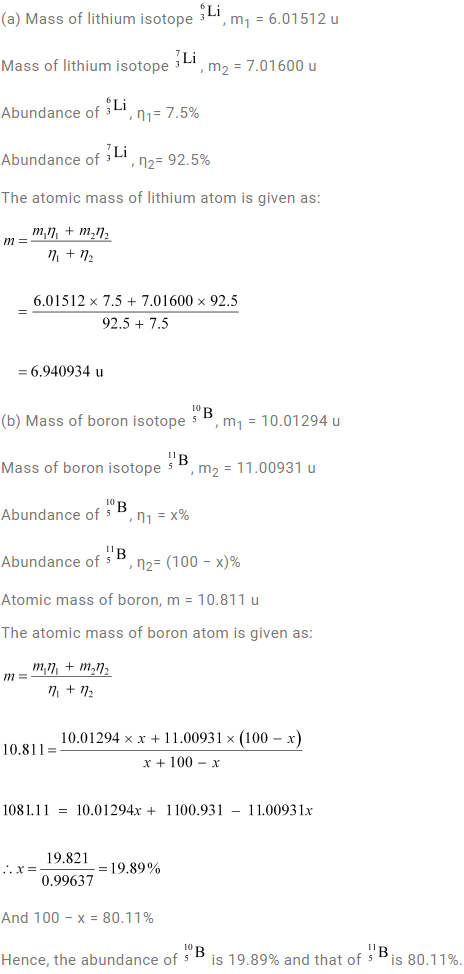Question 13.2: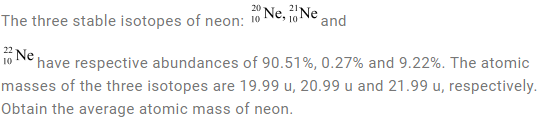Solution: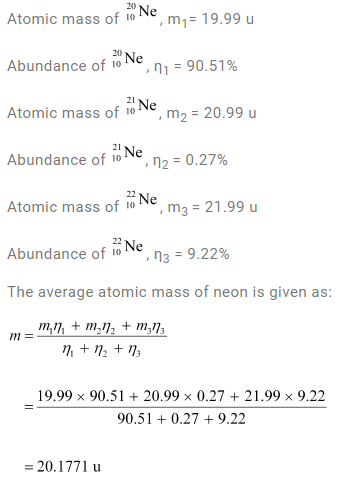Question 13.3: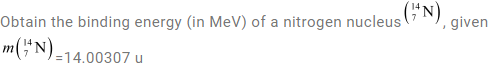Solution: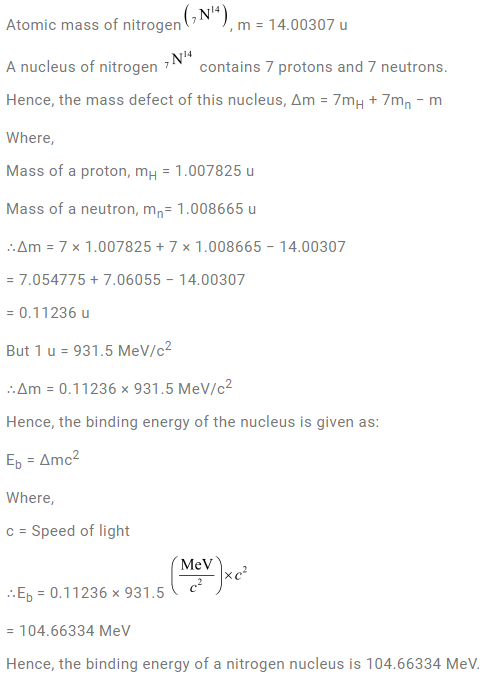Question 13.4: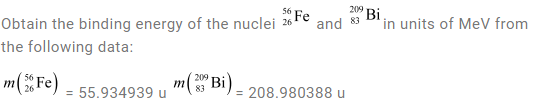Solution: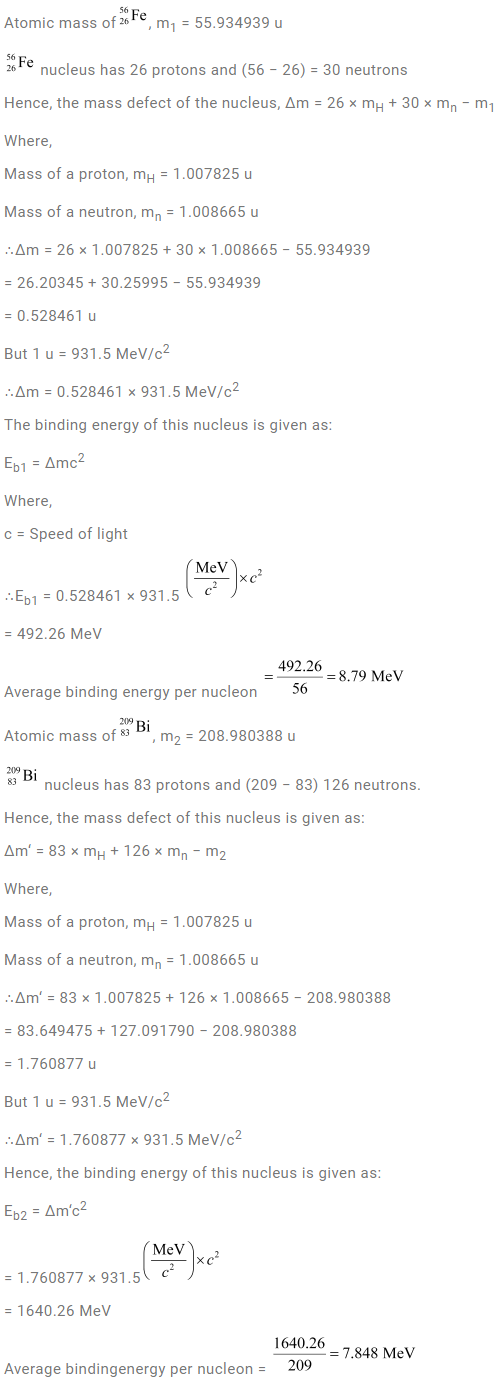Question 13.5: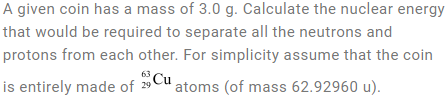Solution: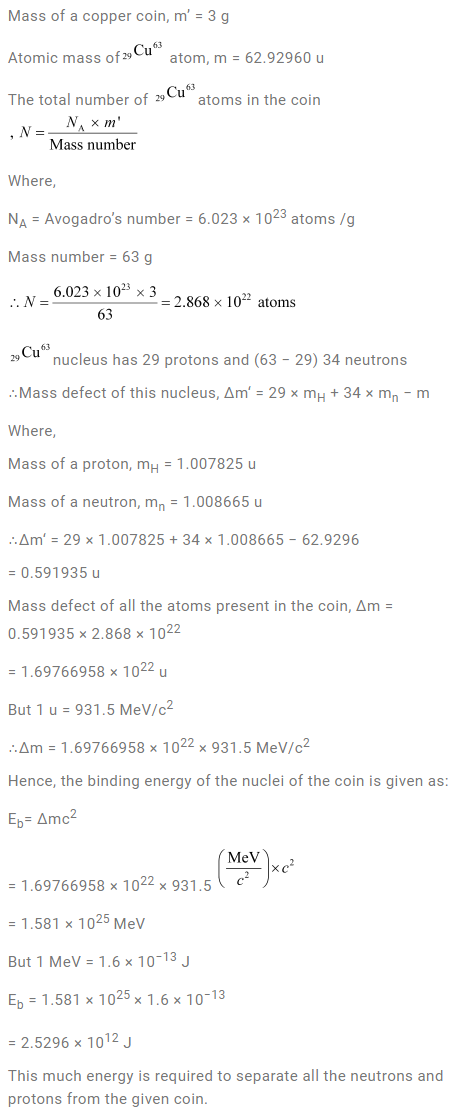Question 13.6: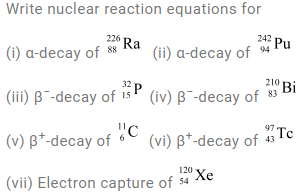Solution: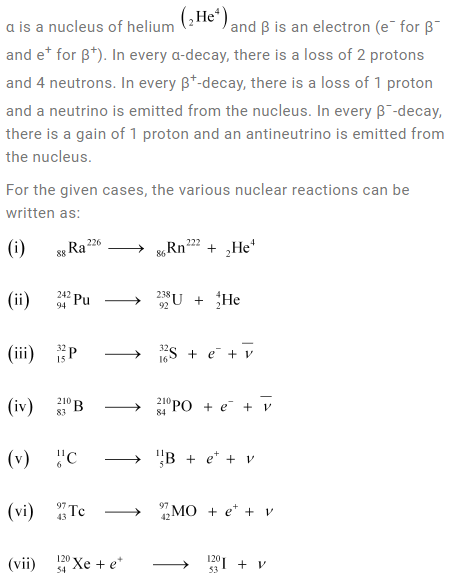Question 13.7: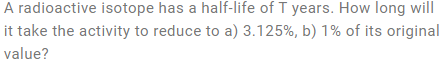Solution: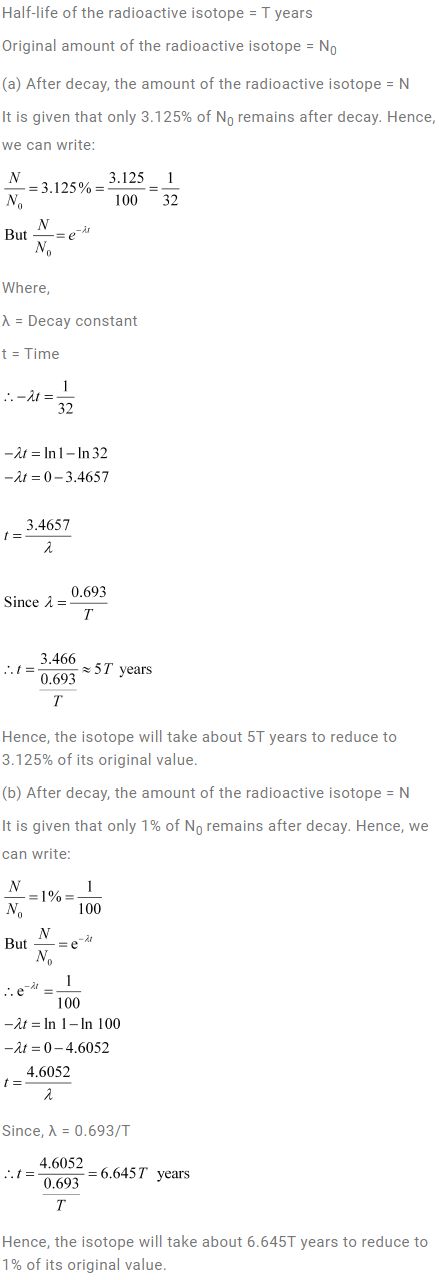Question 13.8: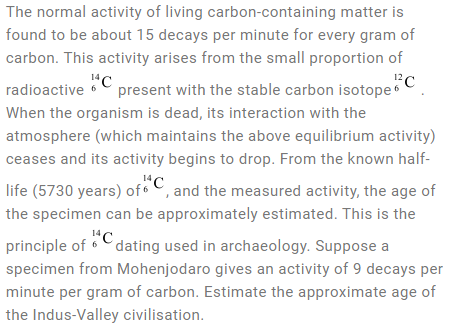Solution: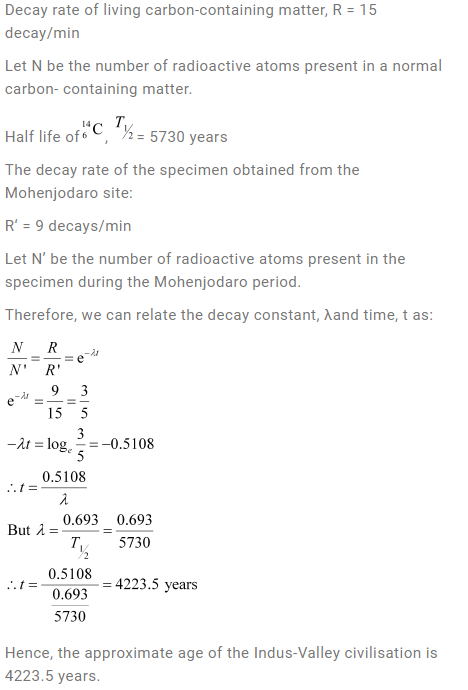Question 13.9: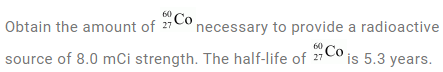Solution: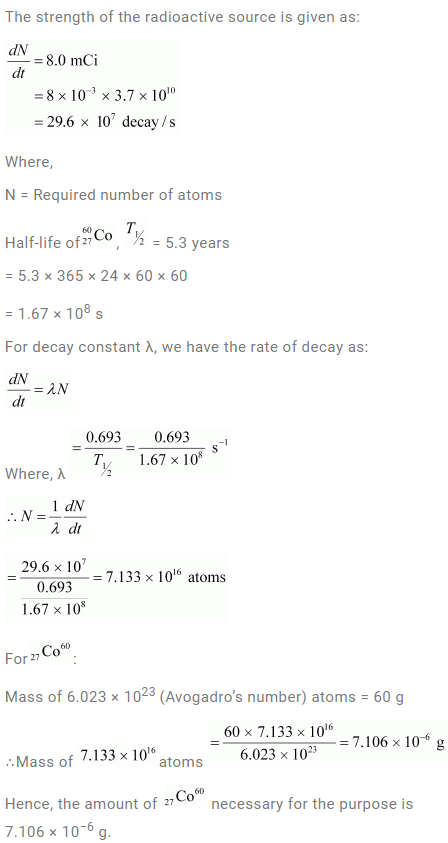Question 13.10: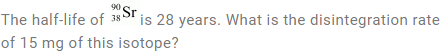Solution: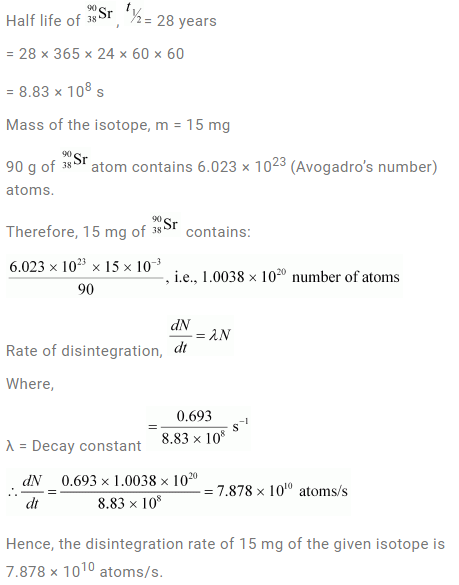Question 13.11:Solution: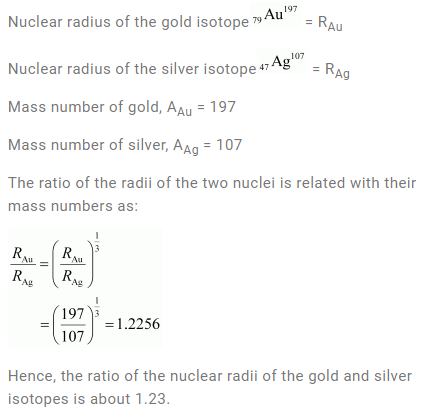Question 13.12: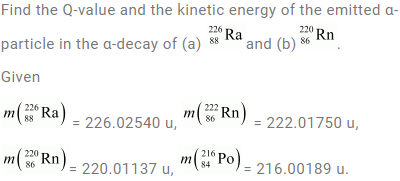Solution: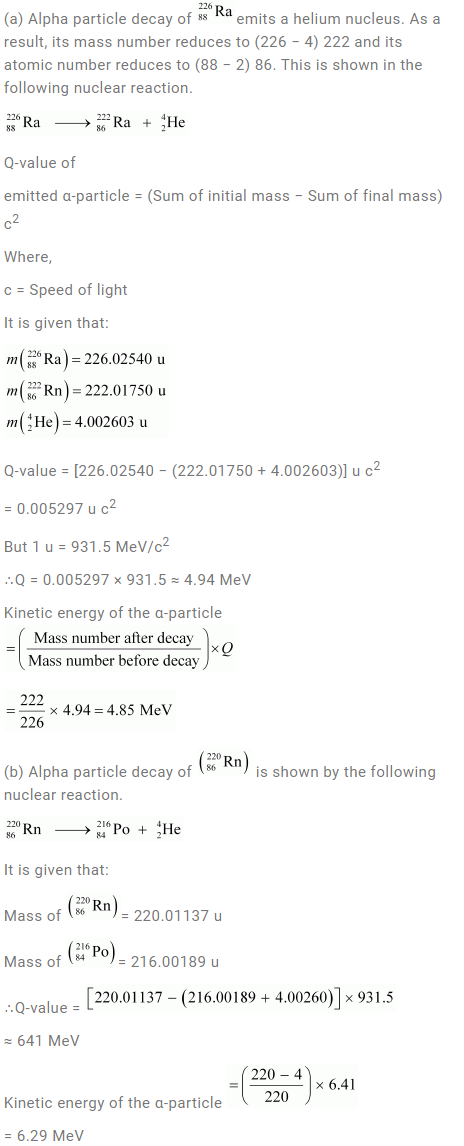Question 13.13: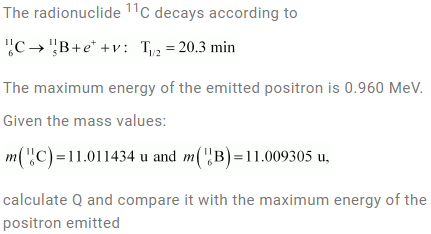Solution: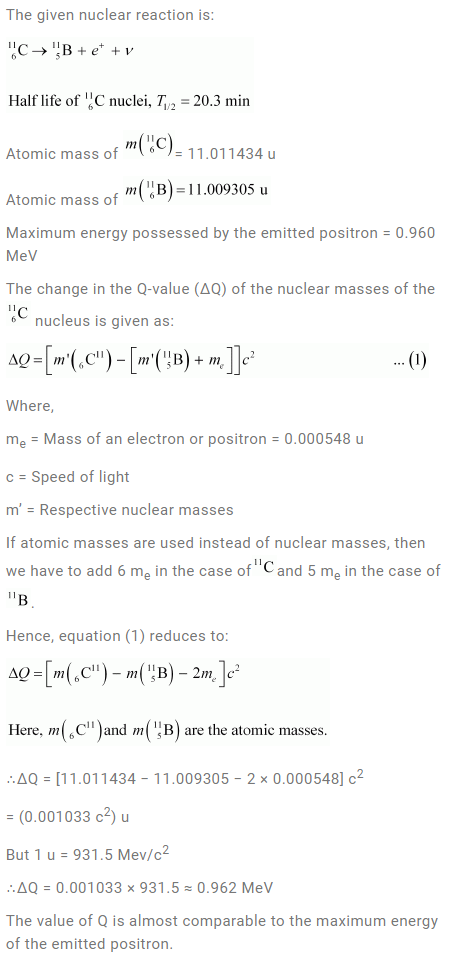Question 13.14: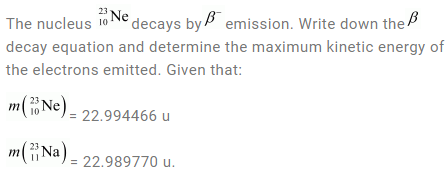Solution: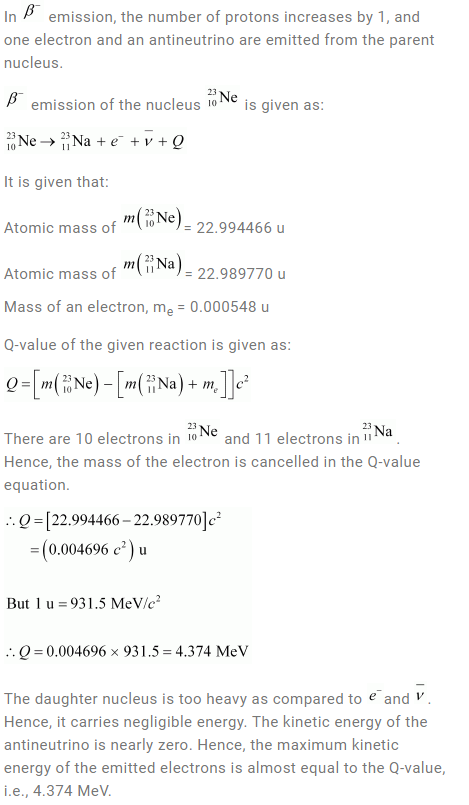Question 13.15: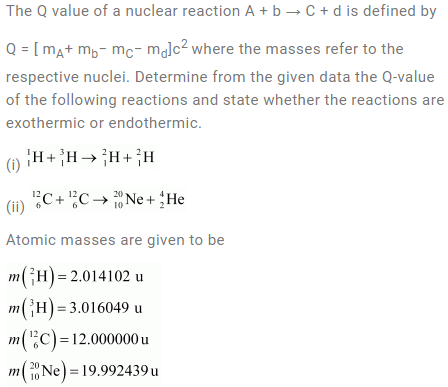Solution: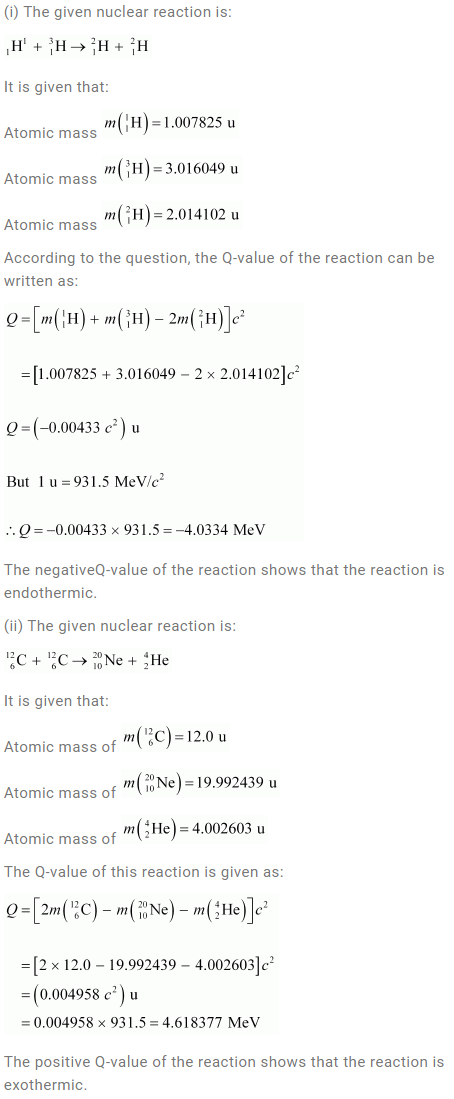Question 13.16: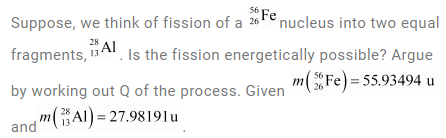Solution: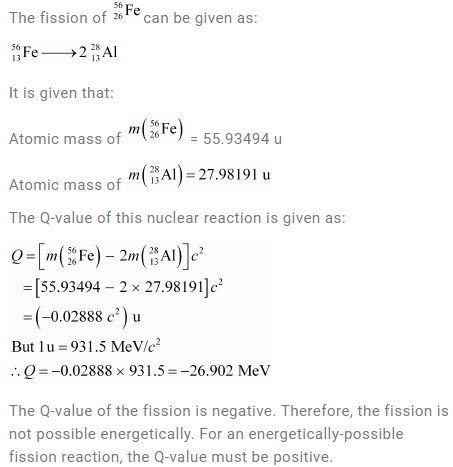Question 13.17: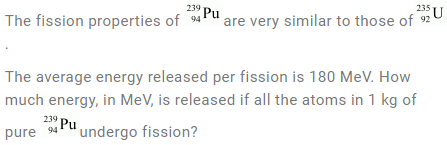Solution: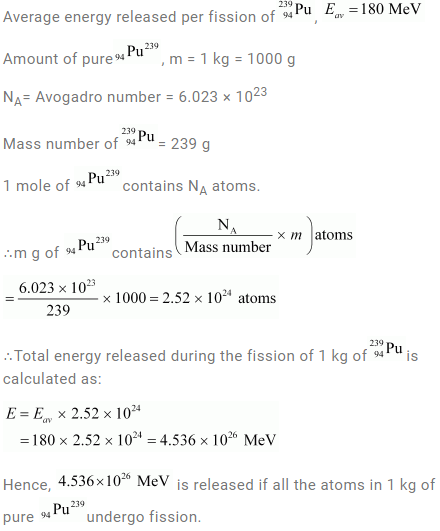Question 13.18: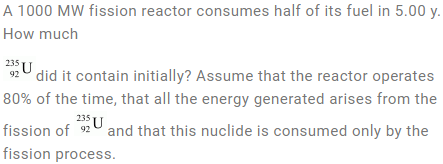Solution: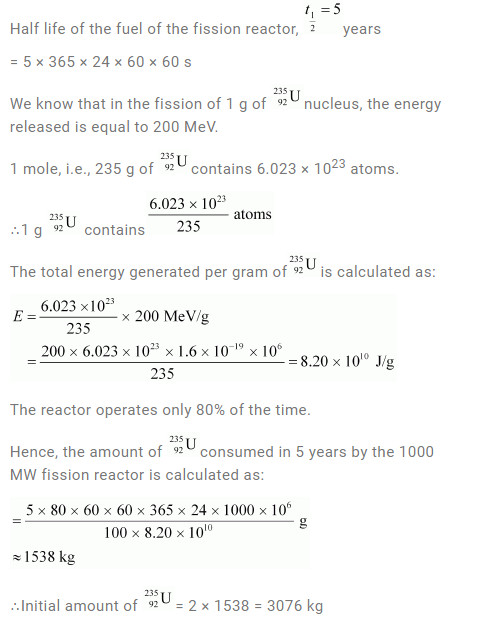Question 13.19: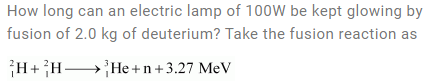Solution: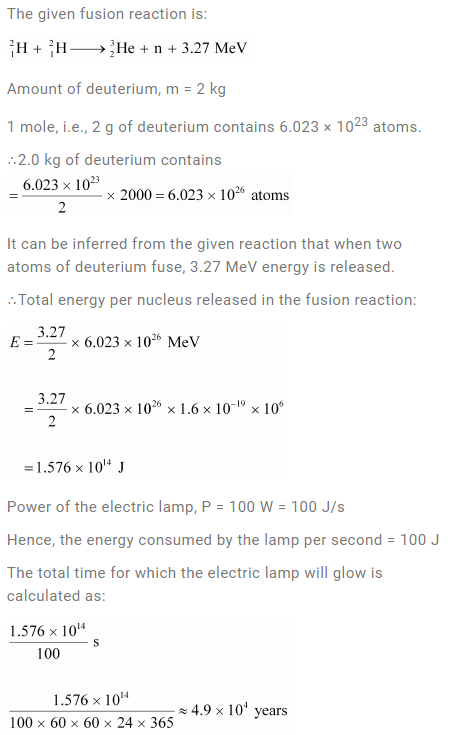Question 13.20: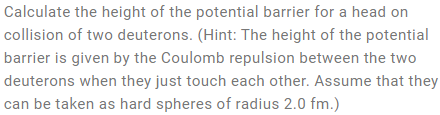Solution: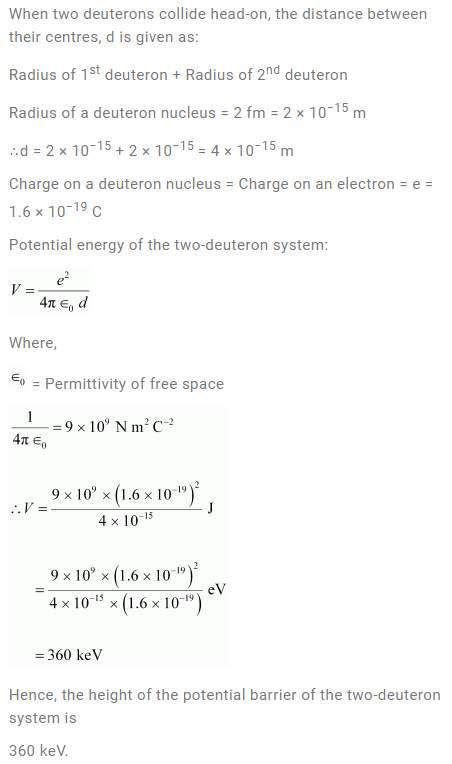Question 13.21: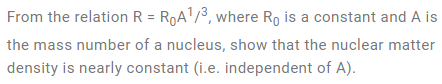Solution: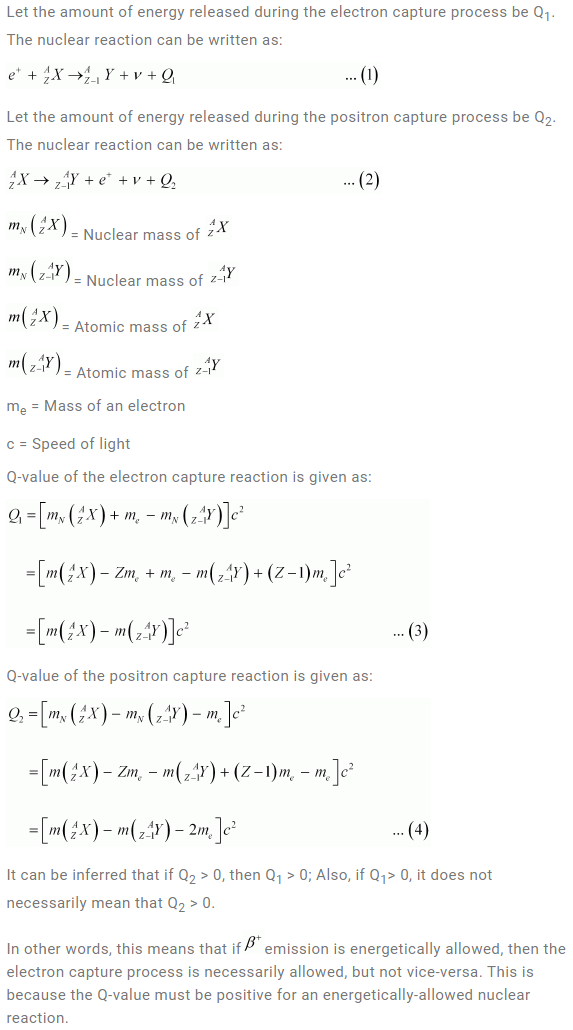Question 13.22: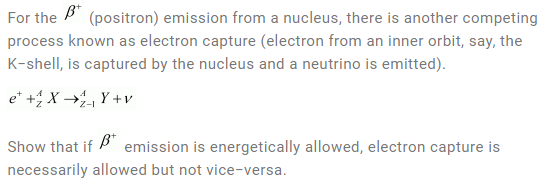Solution: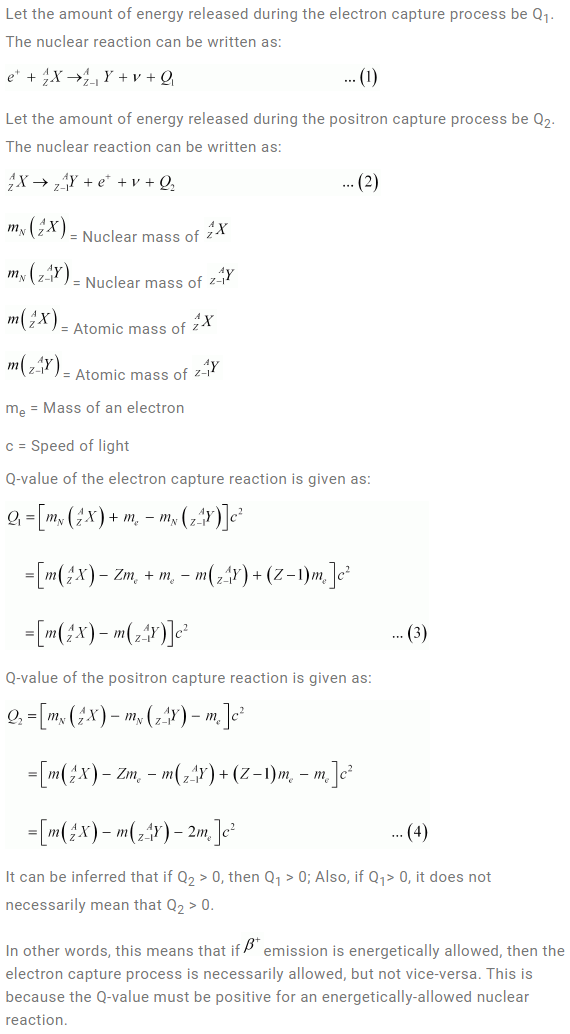Question 13.23: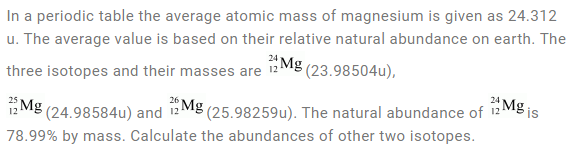Solution: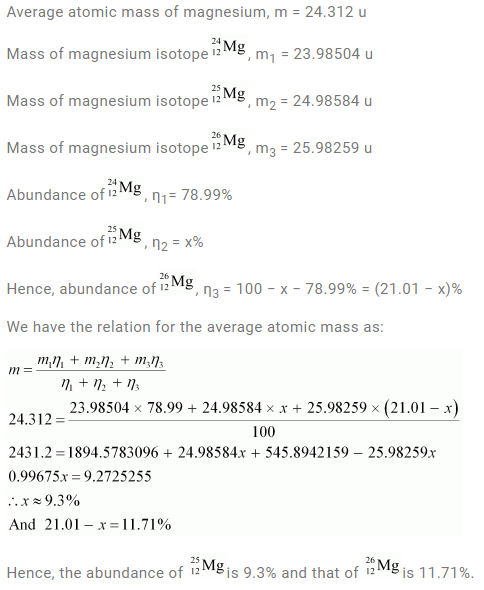Question 13.24: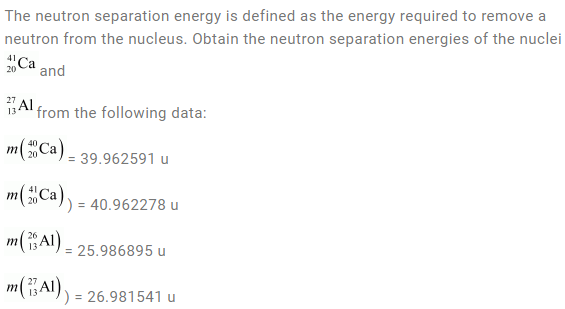Solution: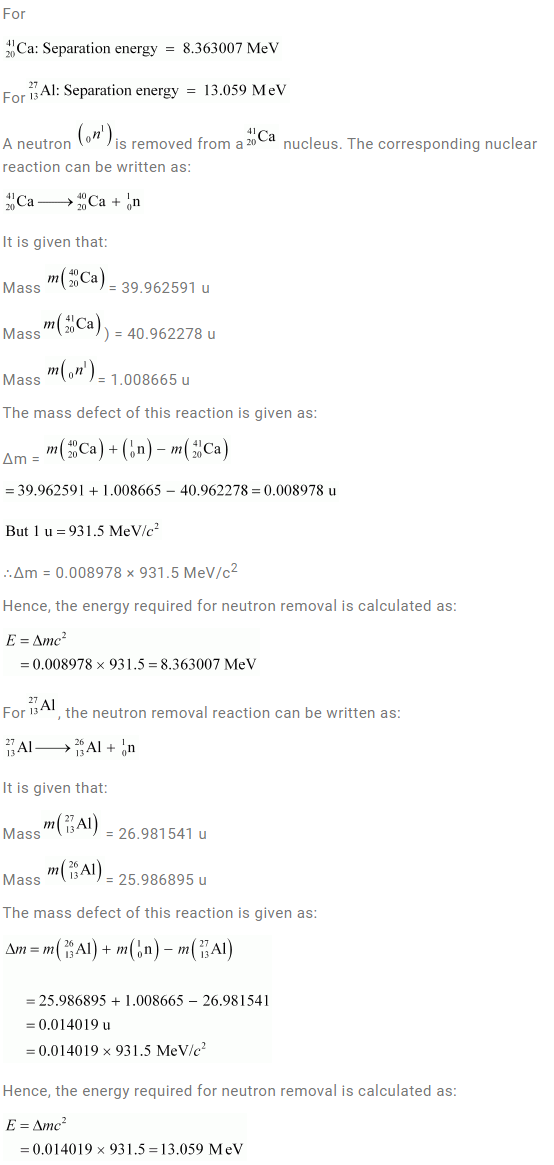Question 13.25: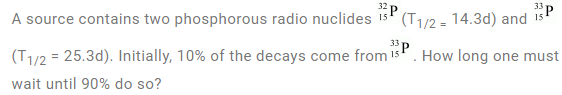Solution: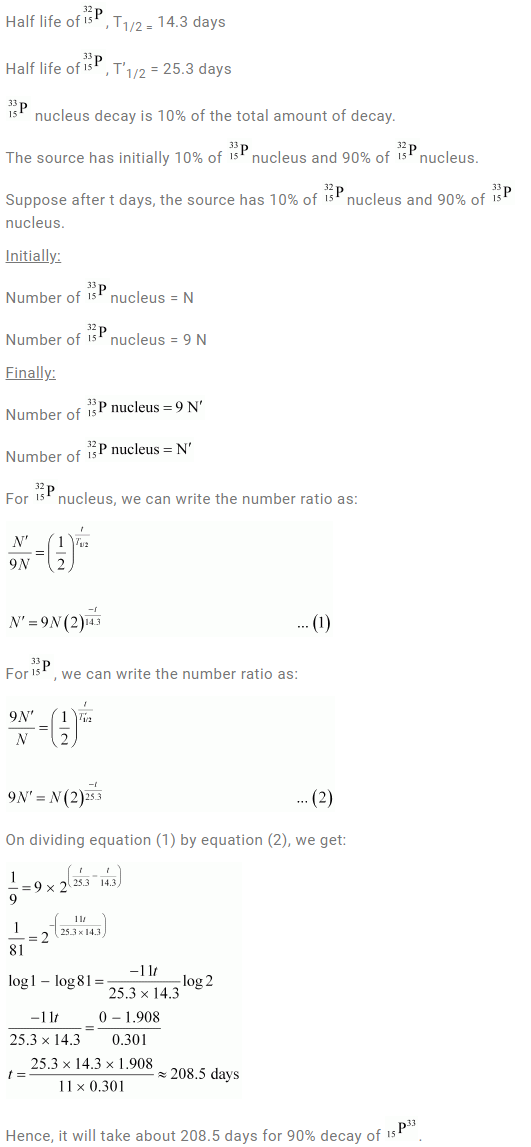Question 13.26: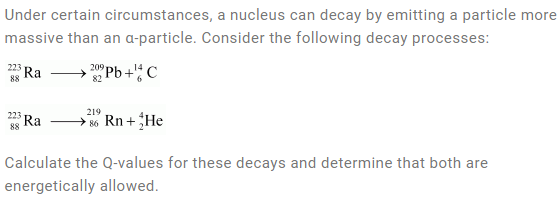Solution: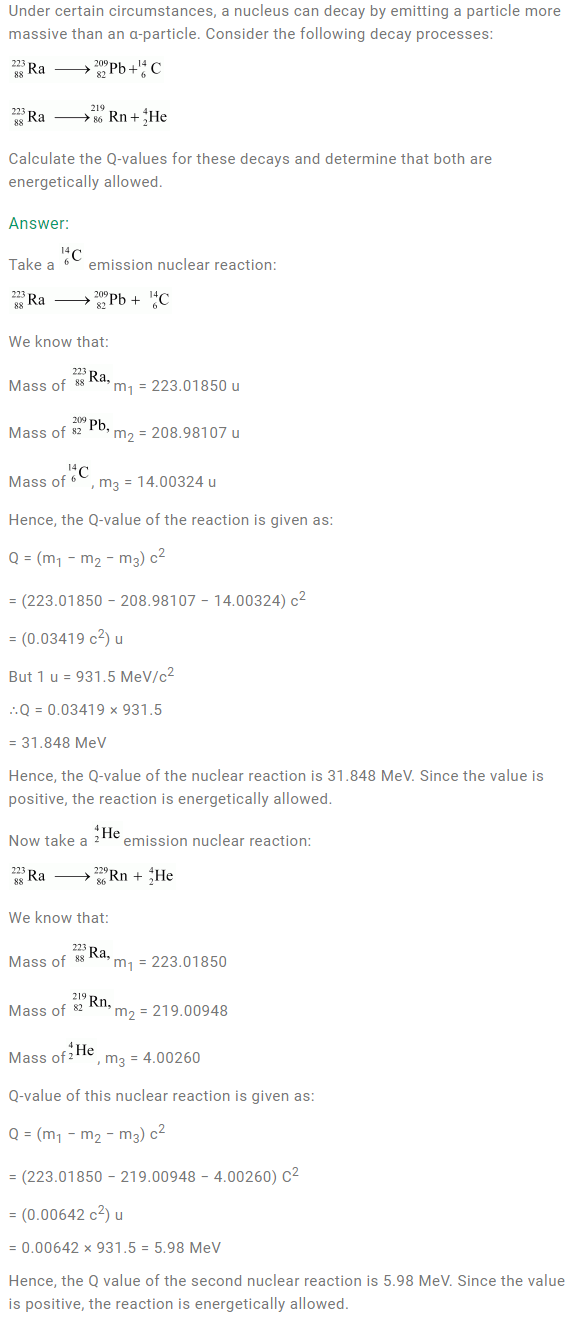Question 13.27: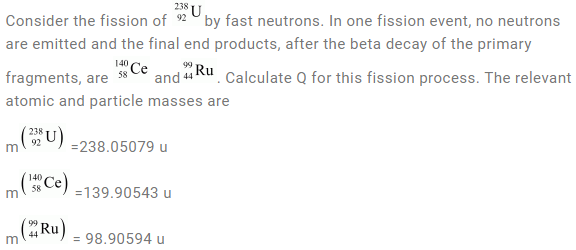Solution: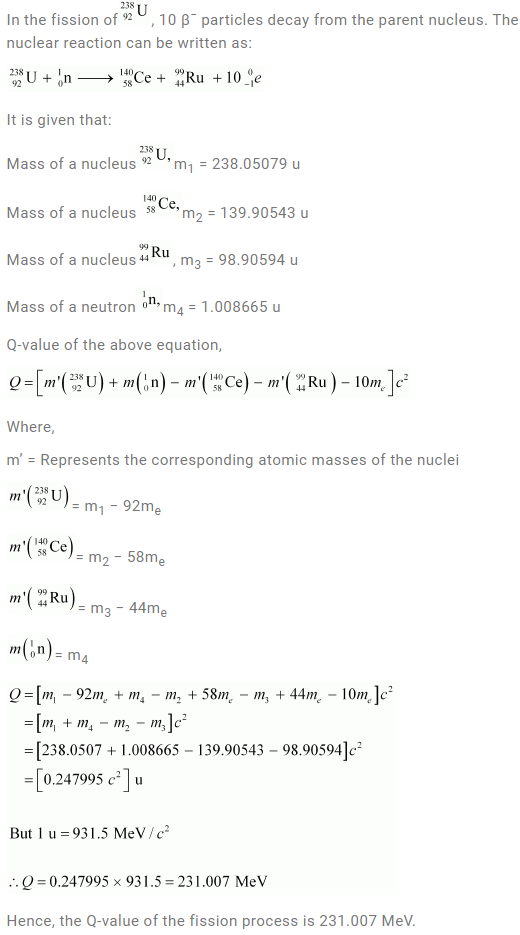Question 13.28: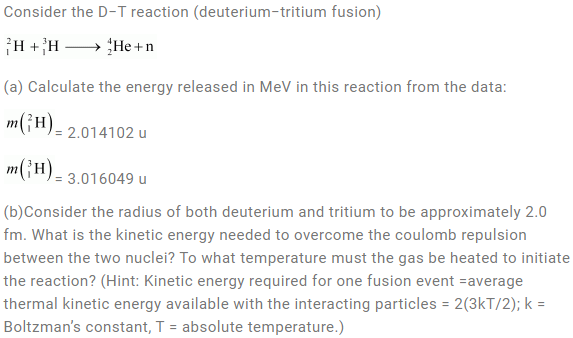Solution:Question 13.29: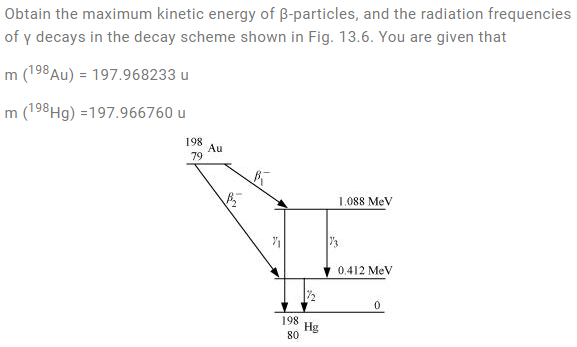Solution: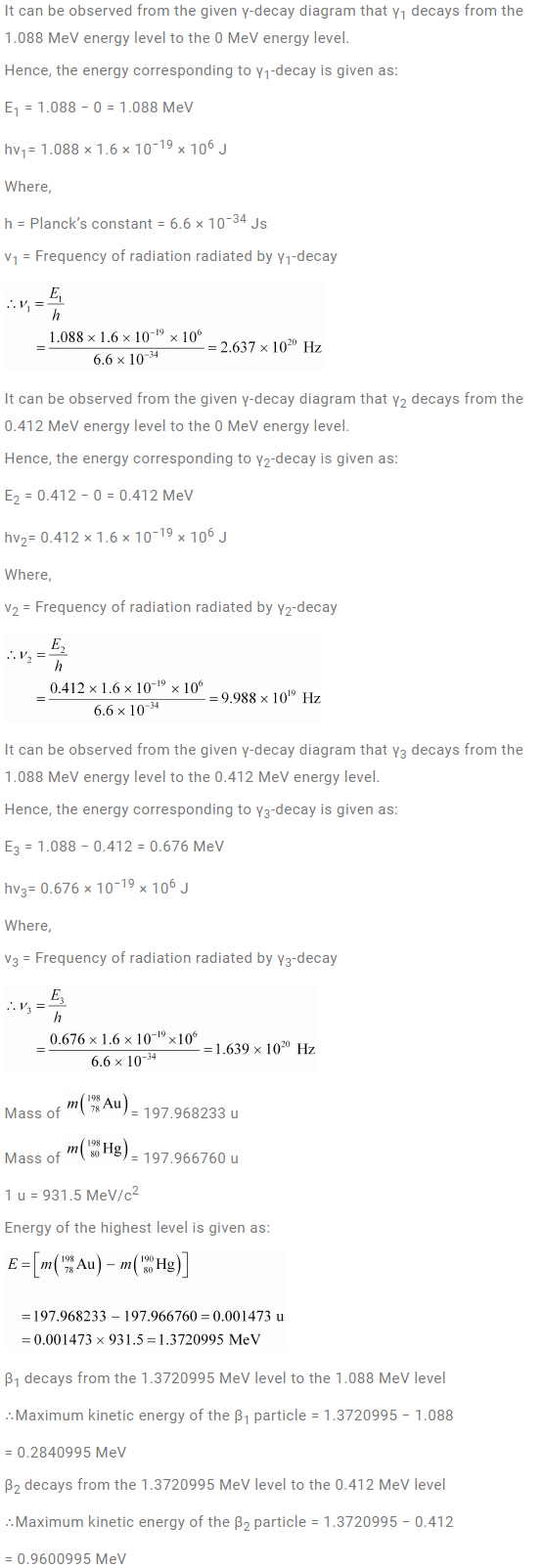Question 13.30: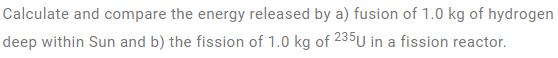Solution: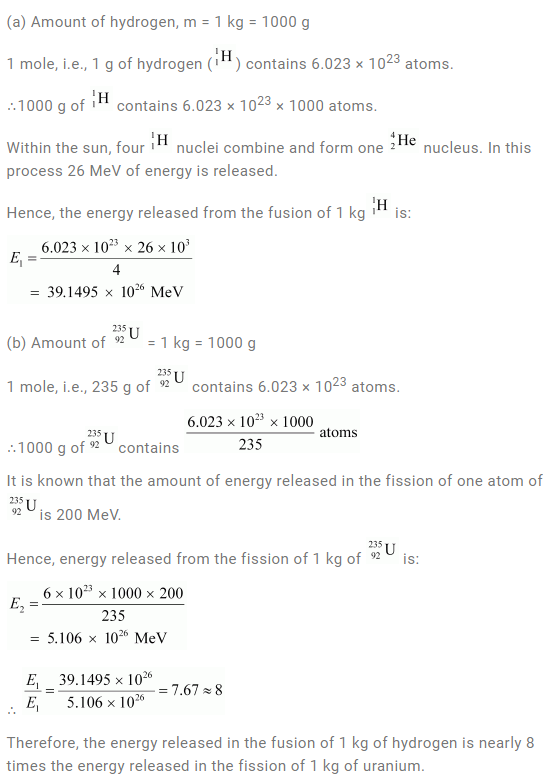Question 13.31: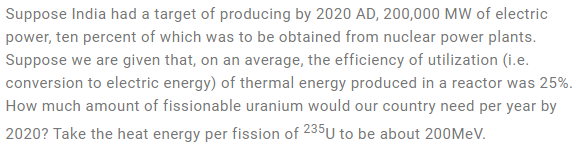Solution: